Intro to Programming: Booleans, Comparison Operators and Logical Operators in Python

In the newest article in our Intro to Programming series, we’ll talk about booleans, comparison operators, and logical operators in Python.
By Ciprian Stratulat • Jan 11, 2022

Welcome back to our Intro to Programming series. Today we’ll talk about booleans, comparison operators, and logical operators in Python.

What are Booleans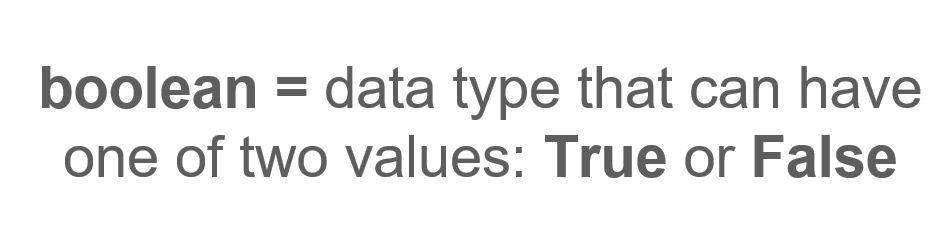By definition, a boolean is a data type that can have one of only two possible values: true or false. Booleans are really important for writing computer logic, and they're used to convey whether something (a statement, a variable, a condition, or other) is either true or false.Booleans get their name from an English gentleman named George Boole. Boole introduced Boolean algebra in his 1847 book entitled The Mathematical Analysis of Logic. If you're not familiar with Boolean algebra, it's a branch of math that operates not with numbers, but with just two values: true and false. In Boolean algebra, true and false are often represented by the numbers 1 and 0, respectively. Though simple on the surface, Boolean algebra can be very powerful. However, we won't go much deeper into the theory behind it. In computer programming, we're more concerned with its applications, and we'll see them more and more as we progress with this course.As you might have guessed, booleans help computers understand logic. By allowing us to assign objects, statements, conditions, or other items a value of true or false, booleans make it possible to execute different parts of a given program by an object’s assigned true or false value.For example, let's consider the statement “Today is December 31”. Depending on when you're reading this post, this statement is either true or false. If today is indeed December 31—first of all, I admire your dedication. Second, you would assign this statement a value of true. Because we can assign a value of either true or false to this statement, a computer can also evaluate it and make a decision based on that statement’s value. The computer can “decide”, for example, to show you a “Happy New Year” message if the statement value is true, or to show you no message at all if it's false.

Booleans as True or False Values

So, we can already see that booleans are essential when we want to execute a part of our program only when a certain condition is true or false. We'll see exactly how that's possible in upcoming articles, but for now that's all you need to remember.
As usual, let's look at some code.

Let's create a variable called is_dec_31. We want this variable to store True if the day is indeed December 31 and False otherwise. Let's say today is indeed December 31. In this case, we can type is_dec_31 = True. If we check the data type of this variable using type(is_dec_31), we get bool, which is short in Python for boolean.

# Let's create our variable
is_dec_31 = True

# and let's check our data type
type(is_dec_31)

# When we run this, we will get bool as the output

But what if today is not December 31? In that case, we can make is_dec_31 = False. We can again check the data type by running type(is_dec_31), and we get bool again.

# Let's change our variable's value
is_dec_31 = False

# Now let's see what data type we have
type(is_dec_31)

# This still returns bool, so we still have a boolean.

One “gotcha” to be aware of is that boolean values in Python need to be capitalized. If, for example, I write is_dec_31 = true, I get an error. The Python interpreter thinks that this lowercase true is the name of a variable, but it can't find any variable by that name. So remember, when assigning True or False boolean values, use capitalization. True with a capital T and False with a capital F are the only reserved Python keywords for boolean values.

# If we don't create our boolean with a capital T or F, we get an error
is_dec_31 = true

# This will return the following error:
#NameError Traceback (most recent call last)
#<ipython-input-7-0e9132fa5c15> in <module>()
#----> 1 is_dec_31 = true
#NameError: name 'true' is not defined

Booleans Evaluating Conditions and Statements

When writing code, you'll rarely work with True and False values directly. Instead, you'll find them as a result of evaluating conditions or other statements. For example, I can write 1 < 2, which is a mathematical expression that also happens to be valid Python code. When I run this, I get the boolean value True, because 1 is indeed less than 2. In fact, you can check that this expression returns a boolean by running type(1 < 2). Doing so returns bool.

# Let's use a boolean to check an equation
1 < 2
# The output will be True

# Let's check the data type of this equation
type(1 < 2)
# Our output will be bool

Conversely, you can also ask Python if 1 > 2. Doing so will return the boolean value False. If needed, you can actually assign that result to a variable. Let's create a variable called condition_is_met and set it to 1 > 2. If we output condition_is_met, we see that its value is False. We know that's because 1 is not greater than 2. Remember that during variable assignment, the Python interpreter first evaluates the expression to the right of the equal sign, which in this case is 1 > 2. In this example, we’ve determined the evaluation of this expression is False, which Python then assigns to the given variable. The data type of the condition_is_met variable is a boolean, as we can verify by running type(condition_is_met).

# First, let's check our equation
1 > 2
# Our output will be False

# Now let's create a variable for a condition
condition_is_met = 1 > 2
#and run the code
condition_is_met
# Our output will be False

# If we check our data type for our variable
type(condition_is_met)
# We will get bool, since it is either True or False

As I mentioned earlier, in most cases you won't directly assign the boolean value True or False to a variable. Instead, you'll assign your variable the result of evaluating a boolean expression or condition, which happens to be either True or False.
That's all on booleans for now. Next, we'll cover comparison operators. We already introduced one here, which is the “greater than” sign. We used it to compare two integer numbers. Python defines several such operators that allow us to test whether two objects are the same, are not the same, or whether one object is less than or greater than another object by some given definition.

Comparison Operators

In past Intro to Programming articles, we’ve learned about the basic data types in Python. Now, it's time to start exploring how to solve problems using computer programs. To do that, we need to learn how to write computer logic. Are you ready? Let's go!

Our first step is a continuation of our boolean discussion. Namely, we’ll talk about comparison operators. Comparison operators don’t come with a lot of theory behind them at this point, so we're going to jump straight into some code.

We've already seen some of these operators, but in this article, we'll go over all of the ones available to us in Python.

Less Than, or <

To start, we saw previously that we can use the “less than” sign to compare two integers in Python. So, if we write 1 < 2, Python returns the boolean value True, since 1 is indeed less than 2. If we input 2 < 1, however, Python returns False. By the way, this operator works with strings, too. For example, inputting 'a' < 'b' returns True because 'a' comes before 'b' in alphabetical order. We can use multiple character strings as well. For example, 'water' < 'air' returns False, because the first letter 'a' comes before the first letter 'w' in the alphabet.

# If we put in a correct equation, we get True
1 < 2
# Our output is True

# When we try an incorrect equation, we get False
2 < 1
# Our output is False

# We can use < with strings, working in alphabetical order
'a' < 'b'
# Our output is True

'water' < 'air'
# Our output is False, since 'w' comes after 'a'

When using comparison operators, pay attention to the two operands. Operands are the things we’re comparing using our operators, and they need to be of the same data type. For example, inputting 2 < '2' will throw an error because we’re comparing an integer number on the left to a string on the right. Python doesn't support that. We can, however, compare two strings as we saw before, even if the strings only contain digits in them. For instance, '1' < '2' returns True. And it's important to understand why this is true: it's not because number 1 is less than number 2. Remember that these are both strings. Instead, Python interprets this expression as True because, alphabetically, the string that contains the character 1 comes before the string that contains the character 2.

# If we try to compare an integer with a string
2 < '2'
# We get the following error:
#→---------------------------------------------------------------------------
#TypeError Traceback (most recent call last)
#<ipython-input-6-cbf4b92c1911> in <module>()
#----> 1 2 < '2'
#TypeError: '<' not supported between instances of 'int' and 'str'

# We can compare two strings with integers
'1' < '2'
# Our output is True because alphanumerically, 1 comes before 2

Another small “gotcha” when comparing strings is that capitalization matters. So, for example, 'Air' < 'air' is True because, in Python, capital letters come before lower-case letters.

# When we run
'Air' < 'air'
# Our output is True

Greater Than, or >

Similar to “less than”, Python also has a “greater than” operator. Everything I’ve said about “less than” also applies to “greater than”. For example, in the simplest form, you can use “greater than” to compare numbers. 2 > 0 returns the boolean value True. Similarly, inputting 'a' > 'b' returns False because ‘a’ comes before ‘b’ alphabetically. Again, you must make sure that the two operands are of the same data type. You can’t use the “greater than” operator to compare strings with integers, for example. To recap, the objects being compared are the operands and the sign used to compare them is the operator.

# We can use the greater than symbol as well
2 > 0
# Our output will be True

'a' > 'b'
# Our output will be False

Equal to, or ==

To check for equality, we can use two equal signs next to each other, like so: 1 == 1. This expression returns the boolean value True. Let's try another one. 1 == 2 returns False. Why? Because 1, obviously, is not equal to 2. We must use two equal signs instead of a single equal sign because Python reserves the single equal sign for variable assignment. To Python, writing 1 = 2 indicates that you're actually trying to assign the value 2 to the integer number 1. Notice that this 1 here is not a variable, but an integer value. But you can't redefine the value of 1, so this expression yields an error. More specifically, Python returns a syntax error.

# We use two equals signs, ==, to show equality
1 == 1
# Our output is True

# If we try to only use one sign
1 = 2
# We get the following error:
#   File "<ipython-input-15-c0ab9e3898ea>", line 1
#1 = 2
#ˆ
#SyntaxError: can't assign to literal

Beginner coders occasionally get confused by this. In the beginning, it's easy to confuse the single equal sign with the double equal, and this will cause the Python interpreter to complain. So, remember that one equal is for assignment, and two equal signs are for comparison.

We can also use this operator to check if two strings are equal. For example, we can check if lowercase 'air' is equal to lowercase 'air' by writing 'air' == 'air'. And, of course, it is. But remember that capitalization matters for strings. You may hear programmers often say that strings are case sensitive. So, if we compare the capitalized string 'Air' with the lowercase 'air' by writing 'air' == 'Air', we'll get the boolean value False because the two strings are not the same. For two strings to be the same in Python, they must have the exact same characters, capitalization and all.

# We can check if strings are equal
'air' == 'air'
# Our output is True

'Air' == 'air'
# Our output is False, because Python is case sensitive

Again, be careful about data types. If we check if the integer number 1 is equal to the string that consists of the character 1, we'll get a False. That's because one of the operands is an integer and the other is a string. A number cannot be equal to a string.

# Pay attention to data types
1 == '1'
# Our output is False because we have a number and a string

You can also use the == operator to compare lists. According to Python, two lists are equal if they contain the same items and those items are listed in the same order. This requirement exists because lists are ordered sequences. For example, in Python the list [1,2] is equal to the list [1,2]. These lists have the same items and the items are in the same order. In contrast, in Python the list [1,2] is not equal to the list [2,1]. Even though these two lists have the same items, their items are in a different order. In Python, the second list is considered a different list.

# Let's compare lists
[1,2] == [1,2]
# Our output is True because the lists have the same items in the same order

[1,2] == [2,1]
# Our output is False because the items are not in the same order

What about dictionaries? We know from previous posts that unlike lists, dictionaries are not ordered. As long as dictionaries have the same pairs, the order in which the pairs are typed doesn't matter to the Python interpreter. For example, if we input {'a': 1, 'b': 2} == {'b': 2, 'a': 1}, Python will return the boolean value True because these notations between each set of curly brackets essentially represent the same dictionary. But, inputting {'a': 1, 'b': 2} == {'a': 1, 'b': 3} will return False. While these two dictionaries do have the same keys, their values are different. Notice that in the second dictionary the string key 'b' is mapped to the integer number 3, not 2.

# Let's compare some dictionaries
{'a' : 1, 'b' : 2} == {'b' : 2, 'a' : 1}
# Our output is True because dictionaries aren't ordered

{'a' : 1, 'b' : 2} == {'a' : 1, 'b' : 3}
# Our output is False because the values are different

Comparison operators also work with variables. Let's create a variable a and assign it to the integer number 2. Using comparison operators, we can compare, for example, the value stored in a with the integer 0. To do so, we run a > 0. In this case, Python returns the boolean value True because a stores the integer number 2, and 2 is greater than 0. Trying again, we can ask if the value stored in a less than 1. Running a < 1 will return False because 2 is not less than 1. Trying again one last time, we ask if a is equal to the integer value 3. The answer, of course, is  False. As Python tells us, the integer 2 is not equal to 3.

# Let's try comparing variables
a = 2

a > 0
# Our output is True

a < 1
# Our output is False

a == 3
# Our output is False

In these comparisons, both of the operands can also be variables. Let's try this out. If we have another variable named b and set that equal to 1, we can check if the value we previously stored in a is greater than the value stored in b. To make this comparison, we type a > b. As you might be able to guess, Python returns True because a stores the integer value 2 and b stores the integer value 1 and, of course, 2 is greater than 1. Comparing our variables a and b is not the same as comparing ‘a’ and ‘b’ as two strings. Comparing the two letters as strings in Python reveals that a variable that contains the character 'a' is not greater than the string that contains the character 'b'. This is because the character “a” comes before the character “b” alphabetically.

# Let's add a second variable
b = 1
a > b
# Our output is True, because 2 > 1

# Remember, variables do not use quotation marks
'a' > 'b'
# Our output is False, because these are strings

This again might be a little confusing in the beginning, so it's always a good idea to keep track of the data type of each object you're dealing with. In the first case above, we know that a is a variable name, which actually stores an integer number. But in the second case, 'a' is a string that contains a single character: the letter “a”. Making this distinction is important.

Not Equal, or !=

The next comparison operator we'll cover is “not equal”. This operator will return True if two objects are not equal and False if they're equal, and its notation in Python is !=. A quick example: running 2 != 1 will return True because 2 is not equal to 1. Under the same logic, running 1 != 1 will return False because 1 is, in fact, equal to 1. And again, this operator also works with strings. Running 'Air' != 'air' will return True, because the two strings use different capitalizations. As discussed before, different capitalizations between objects will cause the Python interpreter to see them distinctly.

# Let's look at not equal, or !=
2 != 1
# Our output is True

1 != 1
# Our output is False

'Air' != 'air'
# Our output is True, because one includes an uppercase letter

Less Than or Equal, or <=

Another operator we can use is “less than or equal”, which is written using a less than sign immediately followed by a single equal sign, <=. For example, to use “less than or equal” we write 1 <= 2. In this case, Python returns True, and it does the same when we try 1 <= 1. Like the others, this operator also works with strings: 'air' <= 'water' is True because 'water' comes after 'air' alphabetically. Similarly, 'air' <= 'air' is also True because the two strings are equal.

# Let's look at less than or equal to
1 <= 2
# Our output is True

1 <= 1
# Our output is still True

'air' <= 'water'
# Our output is True

'air' <= 'air'
# Our output is still True

Greater Than or Equal, or >=

Finally, we also have a “greater than or equal” operator. Running 3 >= 0 in Python will return True, and so will 0 >= 0. Notice that for both “less than or equal” and “greater than or equal” operators, a single equal sign comes after the less than or greater than sign.

# Let's take a look at greater than or equal to
3 >= 0
# Our output is True

0 >= 0
# Our output is still True

By now, we’ve gone over all of the comparison operators available in Python. Comparison operators allow us to compare objects, which is a task that we ask computer programs to do all of the time. As always with new concepts, practice is key to mastery. Spend some time playing with these operators on your own and try out different situations.

Logical Operators

In the last two sections, we'll go over logical operators. While the name might seem intimidating, don't worry. In fact, you've already used logical operators when making decisions for yourself. You probably just didn't know them by this name.In this section, we’ll go over the logical operators AND, OR, and NOT.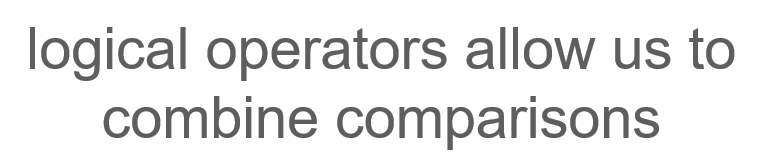Fundamentally, logical operators allow us to combine comparisons, and we use them all the time.For example, if you tell yourself, “I'll go out if it's Friday and the weather is nice”, you're using the logical operator AND to combine two comparisons.Let's break that example down further. The first comparison looks at the current day of the week. As humans, we (usually) store the name of the current day of the week somewhere in our memory. The mechanics of how that information is placed into our memory is irrelevant for our example. Maybe we read it on our phone, or maybe we deduced it by remembering the day of the week it was yesterday. But because the current day of the week is stored in our memory, at any given time we have the ability to evaluate whether the phrase “the current day of the week is Friday” is either true or false.

Perhaps we make our evaluation by comparing the name of the current day of the week with the word “Friday”. If they match, we know that the current day is a Friday. And if they don't, we know that today is not Friday. Of course, when asked, “Is today Friday?” we're able to give an answer immediately. In fact, we're not usually aware of this comparison process as it happens automatically in our minds.

If we were to write this example in computer code, we would probably have a variable called current_day_of_week. In our variable, we’d store a string that tells us what day of the week it is, and we'd compare the value of that variable with the string 'Friday'.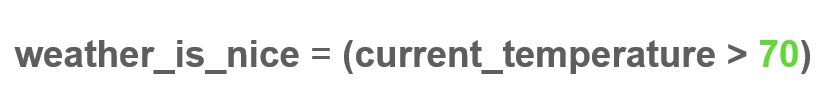The second condition in our combined comparison depends on whether or not the weather outside is “nice”. This condition is more complex because the concept of nice weather is subjective. While most people prefer sunny weather, others may like rain. And even others may get more specific and require their “nice” weather to have a temperature above 70 degrees and below 90. For the purpose of this example, how others personally define nice weather doesn’t matter. Ultimately, the comparison is to see if your definition of nice weather is met. To simplify things, we’ll assume that nice weather means that the current temperature is greater than 70 degrees Fahrenheit.

In code, we can write this comparison using a variable that stores the current_temperature as an integer. Then, we can compare our variable to the integer 70. The current_temperature value may be set by a variety of methods. Maybe the computer reads the temperature from a remote thermometer, or maybe it gets it from the Internet. How it happens doesn't really matter. Once we establish a way to get the value of the current temperature and store it into our variable, we’re ready to combine our comparisons.

Logical Operator And

The logical operator AND allows us to combine our two conditions and follow through with an action only if both of our conditions are met.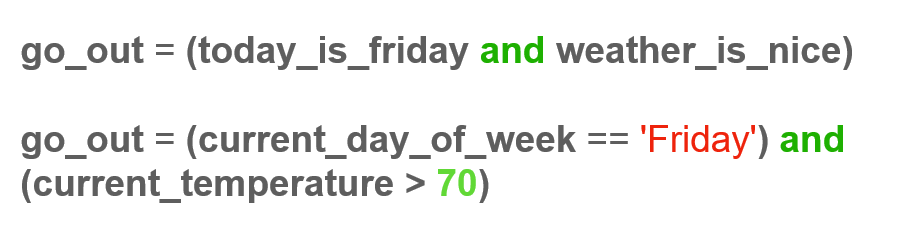In Python, we might write something like go_out = current_day_of_week == 'Friday' and current_temperature > 70.

Here we begin to see the importance of boolean values. Each of these comparisons can be evaluated by Python to equal either True or False, as we learned earlier. But now we can use logical operators to combine those True and False values to get the True or False value for the overall condition we're trying to express. In this example, go_out will store the value True if both current_day_of_week == 'Friday' is True and current_temperature > 70 is True. If either of those conditions equals False, our variable go_out will also be False and consequently, we won't go out.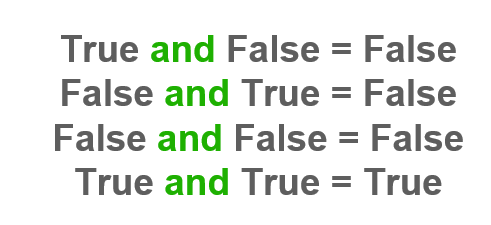Knowing this, we can move on to the truth table for the logical operator AND. A truth table tells us the conditions under which the AND logical operator will return the value True. And from our previous example, we might know intuitively that the only case when the AND logical operator will return True is when all conditions used with AND are true. You can see here that if any of the individual conditions are False, then the overall condition will also be False.

George Boole, by the way, was the first person to formalize the use of logical comparison operators, which is why booleans were named after him. However, humans have been using this kind of logic for a long time before him. Our minds have an amazing complexity that enables us to make logical evaluations implicitly, while also sometimes causing difficulty in expressing our thoughts in easy-to-follow steps. And the latter, in my opinion, makes programming hard for people to learn in the beginning. This difficulty in learning to program does not lie in the syntax or the quirks of specific programming languages. Instead, the difficulty is that we're not used to breaking down our reasoning and expressing it clearly. When we write code, we absolutely have to do this because machines, frankly, are still pretty dumb. In order to complete operations successfully, machines must be told what to do in very small and easy-to-follow steps.

Let's go back to logical operators.So far, we’ve discussed one example where we combined two conditions. But of course, we can also use logical operators to combine as many conditions as a given situation needs. Let's imagine another example. Let’s say that I’ve decided that I’ll meet my friend if it’s after 6pm, and I’m free, and I don’t have a headache. Here, I’m using two AND logical operators to combine three conditions, and I'll only meet my friend if all three of these conditions are True.

Logical Operator Or

The logical operator AND will return True if—and only if—all comparisons or conditions it combines are True.
Let's look at the second logical operator we want to cover in this post: OR.As usual, we’ll start with an example. Take the phrase, “I'll meet with friends on Saturday or Sunday”. In order to determine whether or not we should meet with friends, we need to do two comparisons.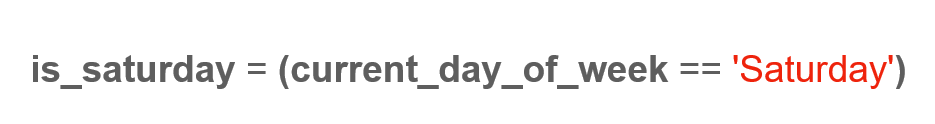The first one compares the current day of the week with the string 'Saturday'.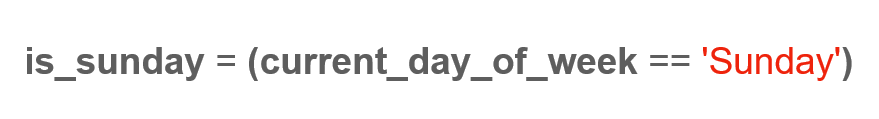The second one compares the current day of the week with the string 'Sunday'.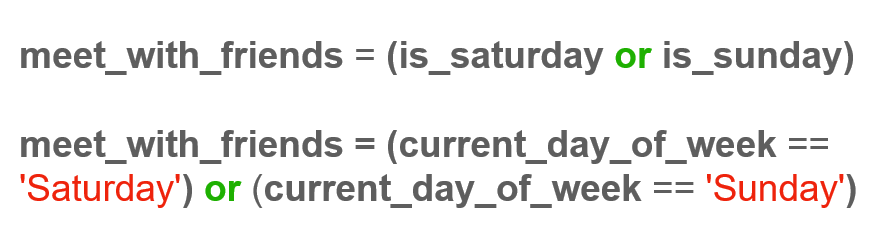To make these comparisons in Python, we can write this as meet_with_friends = current_day_of_week == 'Saturday' or current_day_of_week == 'Sunday'. If any one of these comparisons is True, then the variable meet_with_friends will also store the boolean value True.

The OR logical operator is similar to the AND logical operator in that it combines comparisons and returns a boolean value. The important difference is that while the AND logical operator returns True if all of the combined comparisons are True, the OR logical operator returns True if at least one of the comparisons is True. This, in turn, gives us the following truth table. Similar to the AND logical operator, you can combine as many comparisons or conditions as you want using OR.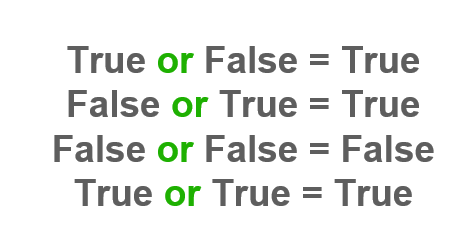Logical Operator Not

Finally, let's briefly talk about NOT. The NOT logical operator is used for negation, as the keyword implies. In other words, NOT is used to express conditions that are met when a comparison is False. For example, let’s look at the following sentence: “The weather is nice if the temperature is not below 70.” We can choose from one of two ways to express this.The first way is to rephrase this sentence to say that the weather is nice when the temperature is greater than or equal to 70. If we think back to the previous example and assume that we have a variable that stores the current temperature, we can write this in Python as weather_is_nice = current_temperature >= 70. This code would definitely match the condition we expressed.

But sometimes rephrasing the comparison in our heads can be more complicated. For those scenarios, we can use the NOT logical operator.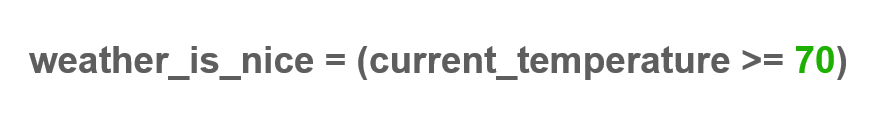In Python, we have the option to use the NOT logical operator to express the original condition of “The weather is nice if the temperature is not below 70” as weather_is_nice = not current_temperature < 70.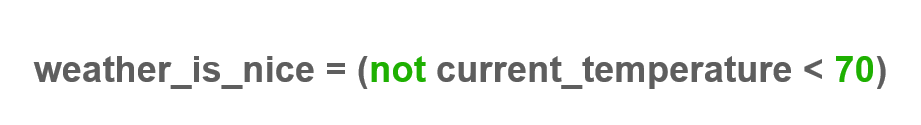The truth table for the NOT logical operator is actually quite simple, and you can see it here. The table shows that NOT True is equal to False and NOT False is equal to True.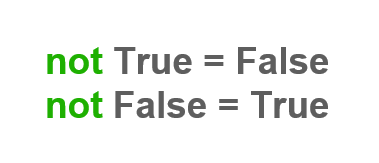I wanted to show with these examples that this process of expressing and evaluating logical operators is not something new at all. In fact, as humans, we can do it easily and we do it naturally all the time. Yet, the ability to express the use of logical operators in computer code is part of why programming is such a powerful tool. A good mental exercise is to take some of the conditions you think of or express daily and write them as comparisons linked by logical operators. For this exercise, assume you have variables available that store important pieces of information. These variables may store current time, current day, or current temperature, to name a few examples. Write your comparisons with logical operators on a piece of paper and see if they make sense.

That's all for the logical operators AND, OR and NOT. In our final section, we'll explore how we can use these logical operators to write Python code.

Logical Operators Code

Let’s take a look at our three logical operators, AND, OR and NOT, in action with a few more examples.

Let's assume that we have a variable that stores the current day of the week. We'll make that variable equal to the string ‘Thursday’.

How to Use Or

Using our variable, we can write the comparison is_weekend = current_day_of_week == ‘Saturday’ OR current_day_of_week == ‘Sunday’. If we check the variable is_weekend, we’ll see that it’s False. That’s because the string 'Thursday' doesn't equal the string 'Saturday', and it also doesn't equal the string 'Sunday'.

# Let's define our variable
current_day_of_week = 'Thursday'

# Now let's write our comparison
is_weekend = current_day_of_week == 'Saturday' or current_day_of_week == 'Sunday'

# Let's check our comparison
is_weekend
# Our output is False

Let’s go back and change current_day_of_week to 'Saturday' and re-run our example.

# Let's update our variable
current_day_of_week = 'Saturday'
# and rerun our example
is_weekend
# Our output is True

We see that now is_weekend stores the boolean value True, because the first of the conditions is true. That is to say that current_day_of_week stores the string 'Saturday'.

A best practice in general is to wrap comparisons in parentheses to organize the code more clearly. I recommend that you do so with your code as well. Following this convention, we'd rewrite our example as is_weekend = (current_day_of_week == 'Saturday') or (current_day_of_week == 'Sunday').

# Let's make our code cleaner
is_weekend - (current_day_of_week == 'Saturday') or (current_day_of_week == 'Sunday')
is_weekend
# Our output is still True, but our code looks better

How to Use And

Let's make this a bit more complex. Let's assume that we can ask a user whether they feel good or bad, and we can store their answer in a boolean variable. Let's call our variable user_feels_good. When the user tells us that, indeed, they feel good, we'll set user_feels_good to the boolean value True. But how would we express the question, “Is it a weekend and does the user feel good?” The answer is pretty simple: we’d type is_weekend and user_feels_good.

Hypothetically, these comparisons could be a condition for meeting with friends. If that’s the case, we can say meet_friends = is_weekend and user_feels_good to store our result into a variable meet_friends. If we print out meet_friends, we see that it's True because the user told us they feel good and it is indeed the weekend. (At least it is in our example, since we set current_day_of_week to be Saturday above.) By the way, we could also express this comparison in a single line as meet_friends = (current_day_of_week == 'Saturday') or (current_day_of_week == 'Sunday') and user_feels_good. Doing so, we can, again, output meet_friends and see that it's still True.

user_feels_good = True

# Let's set our conditions
meet_friends = is_weekend and user_feels_good
meet_friends
# Our output is True

#We could combine these conditions on the same line:
meet_friends = ((current_day_of_week == 'Saturday') or (current_day_or_week == 'Sunday)) and user_feels_good
meet_friends
# Our output is still True

A Note on Parentheses

I want to point out a couple of things here. First, note that we don't need to wrap single boolean variables in parentheses. In our example, we did not use parentheses around user_feels_good. Second, notice how I wrapped everything to the left of and in parentheses. That's because we consider that whole segment of code a condition by itself.

Parentheses are important and where you place them matters because they change the order in which conditions are evaluated. Let me show you why. Let's say I have this condition: (1 == 3 and 1 == 1) or ('a' == 'a'). These conditions will return True because the first condition, 1 == 3 and 1 == 1, returns False, but the second condition, 'a' == 'a' returns True. We know that the logical operator OR will return True if at least one of the conditions is True.

# Let's break these parentheses down:
(1 == 3 and 1 == 1) or ('a' == 'a')
# Our output is True, because while part one was False, part two was True
# The OR here allows this to be True

Compare that with the condition (1 == 3) and (1 == 1 or 'a' == 'a'). Here, we only shifted the parentheses but this condition will return False. Why is that? Remember that Python will always evaluate the code in parentheses first. In this example, if we look at the first set of parentheses that has the single comparison 1 == 3, we see that it is obviously False. The second set of parentheses in our example evaluates to True because an OR will have a True result as long as any of its comparisons is True. In this case, both comparisons evaluate to True. Putting it together, the whole expression evaluates to False AND True, which is ultimately False. The AND logical operator requires all conditions to be True for the result to be True.

# Let's break these parentheses down
(1 == 3) and (1 == 1 or 'a' == 'a')
# This is False, because the first part is False
# The AND makes this False

In this example, the parentheses completely altered the conditions. When using logical comparisons, be careful that you put your parentheses where you actually intend them to be. Otherwise, subtle bugs may creep into your code, and it could take you a long time to find them.

How to Use Not

Finally, let me show you one more example of the NOT logical operator in action. Let's say that we now want to create a user_feels_bad variable that is basically the opposite of user_feels_good. In other words, this variable will indicate that the user feels bad when they don't feel good. I can write that as user_feels_bad = not user_feels_good. So now, if I output user_feels_bad, I see that it's False, and that's because the variable user_feels_good was set to True above.

# Let's add a NOT variable
# Our output is False

Of course, the NOT logical operator can be used to negate much more complex conditions. For example, I’ll write not (1 == 1 or 'a' == 'b'). Let's go over this and break it down. First, we must evaluate what's inside the parentheses first because they have the highest priority, as we saw. Because 1 indeed does equal 1 and the OR logical operator needs at least one of the conditions to be True, the whole phrase within the parentheses evaluates to True. This expression is equivalent to NOT True, which, of course, is False.

# Let's try another NOT example
not (1 == 1 or 'a' == 'b')
# Our output is False because the code inside the parentheses is True

If you're ready for a bit of philosophy, check this out: not (True == False). If we run this, we get True. This is essentially a way of asking the question, “Is it true that truth and falsehood are not the same?” And the answer is, of course, that it is true.

# Let's try our philosophical example
not True
# Our output is False

not (True == False)

So that's it for logical operators. It may feel strange in the beginning to express conditions this way, but practice will make it second nature. As we start writing more complex problems, logical operators will become indispensable. But for now, just remember that we can use logical operators to combine comparisons in order to express more complex conditions. We use the AND logical operator when we want all conditions to be true, and the OR logical operator when we want at least one condition to be true. We use the NOT logical operator to get the opposite truth value, so when we want False instead of True and True instead of False.Ciprian Stratulat

Ciprian is a software engineer and the CTO of Edlitera. As an instructor, Ciprian is a big believer in first building an intuition about a new topic, and then mastering it through guided deliberate practice.

Before Edlitera, Ciprian worked as a Software Engineer in finance, biotech, genomics and e-book publishing. Ciprian holds a degree in Computer Science from Harvard University.